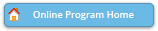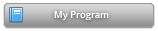#### Abstract Details

 Activity Number: 433 Type: Contributed Date/Time: Tuesday, August 2, 2016 : 2:00 PM to 3:50 PM Sponsor: Section on Statistical Learning and Data Science Abstract #318608 Title: Exponential Random Graph Models with Stable (Nonparametric) Statistics Author(s): Goeran Kauermann* Companies: Ludwig-Maximilians-University Munich Keywords: Network Data ; Exponential Random Graph Model ; Non-parametric Statistics Abstract: Exponential Random Graph Models (ERGM) are a common tool for network data analyses. The ERGM describes the distribution of a network graph with an exponential family distribution, where the statistics are counts of edges, k-stars or triangles, for instance. Even though this allows for simple and meaningful interpretations of network data the models are notoriously unstable if the network is large, i.e. if there are several hundred nodes in the network. To overcome this problem a number of modifications have been pursued, including curved exponential models and geometrically down-weighted statistics, which come however for the price of reduced interpretability. We propose to make use of simple statistics like k-stars or triangles, which allow for intuitive and meaningful interpretations. But instead of using linear forms of the statistics we use non-linear versions, where the latter are either fitted by non-parametric, monotonic splines or by pre-specified curved functions. The approach provides simple interpretations of network data by maintaining stability of the model. Moreover, parallel computing can be employed to estimate the Maximum-Likelihood estimate.

Authors who are presenting talks have a * after their name.